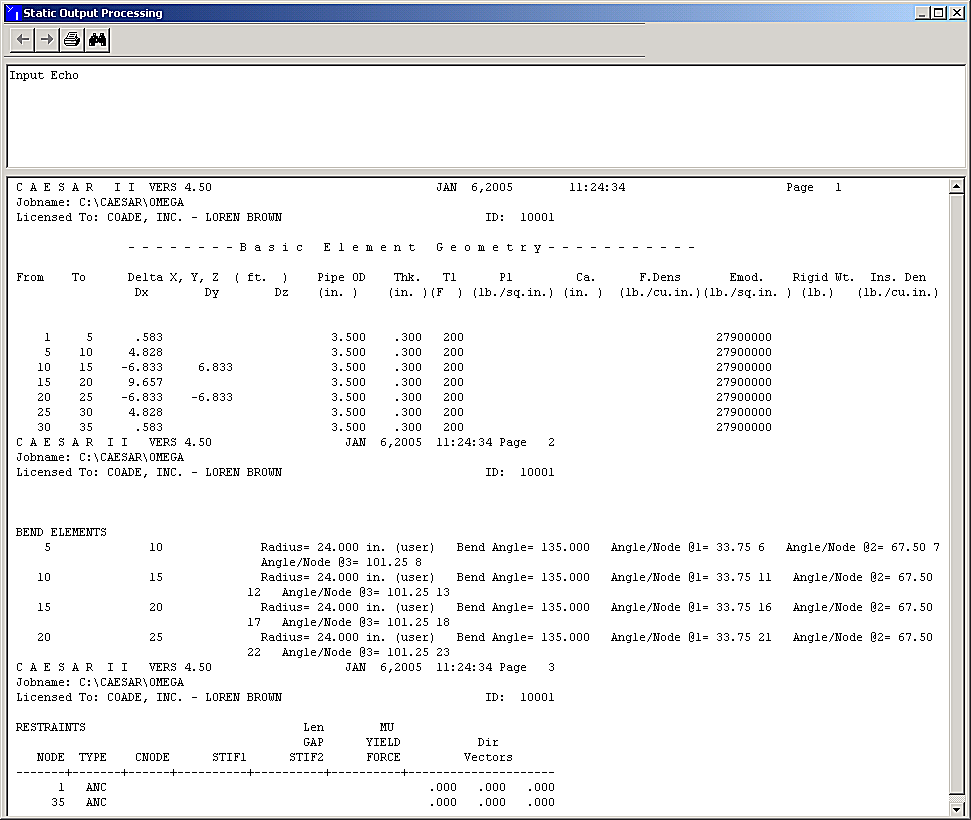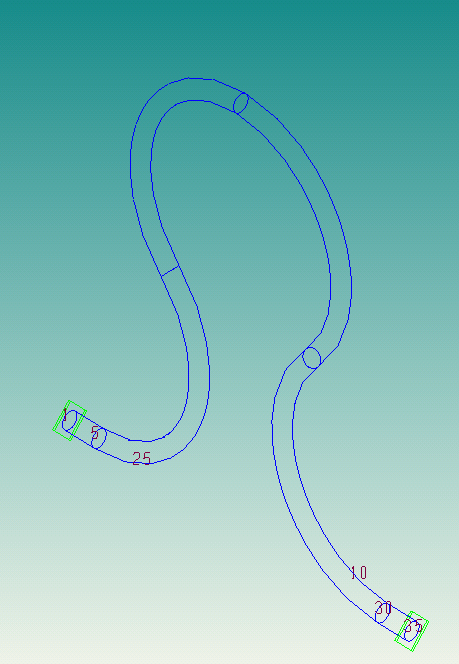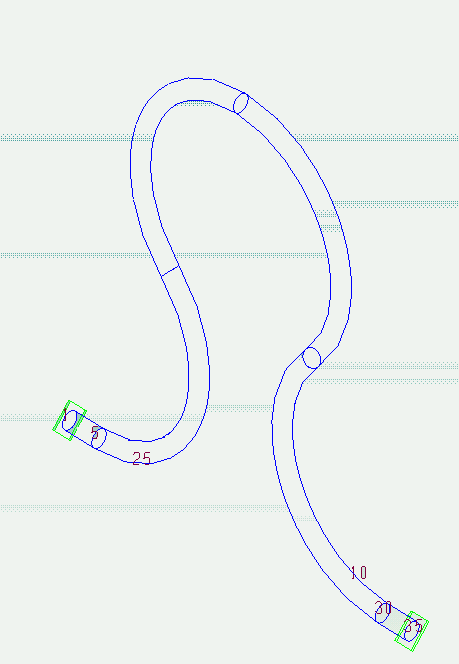# Omega Loop Modelling (OMEGA) - CAESAR II - Reference Data

## CAESAR II Applications Guide (2019 Service Pack 1)

PPMProduct
CAESAR II
PPMCategory_custom
Reference Data
Version_CAESAR
11.0 (2019)

The Omega expansion loop consists of a series of back to back 135 degree bends. This example illustrates how to generate a piping model of an Omega loop according to the following sketches.

• Pipe: 3-inches, standard wall

• Material: Low carbon steel

• Temperature: 200°F, 300°F, 400°F

The example uses an Omega loop as shown below. The given dimensions are the 6-ft 10-in. height, the 2-ft bend radius, and the bend angles of 135° and 270°. Using this information, you can derive the other dimensions shown in the following figure.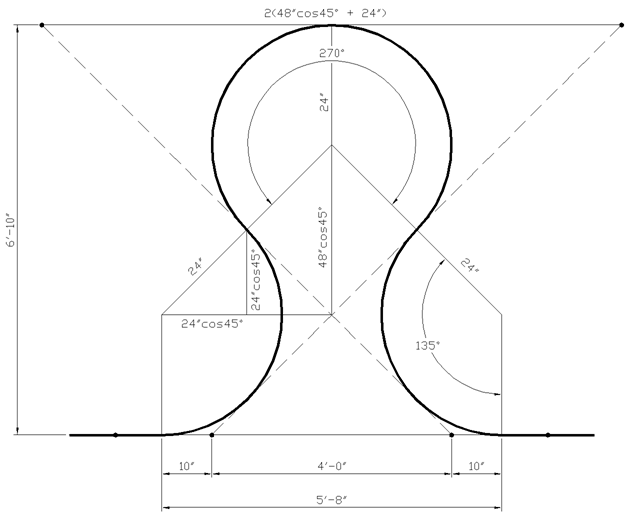Equation 1: Figure 1

When coding a series of back-to-back bends, it is important to remember that the delta dimensions should be measured from the tangent intersection point (TIP) to the tangent intersection point.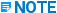For more information about the proper coding of bends, see Bends.

Figure 2 shows the node points, which are coded on the Classic Piping Input dialog box to model the Omega loop. The model is anchored at nodes 1 and 35. The first bend (lower left bend) spans between nodes 5 and 10. The TIP 10 is to the far right of the bend. For analysis and output, the actual location of node 10 is at the far weld line, as shown in Figure 3.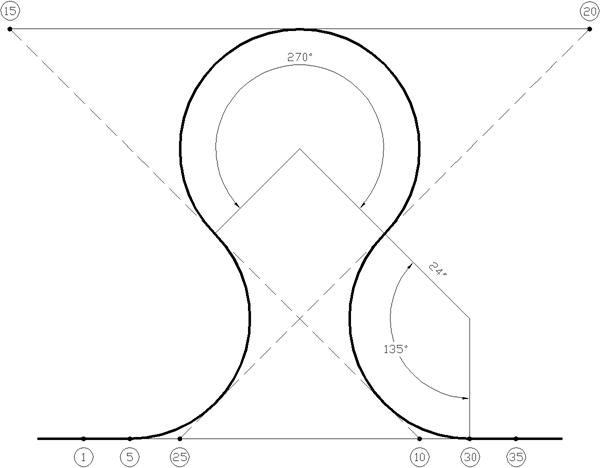Equation 2: Figure 2

The second bend (upper left bend) spans between nodes 10 and 15. Because you can code TIP to TIP, the delta coordinates entered in the Classic Piping Input dialog box are the X and Y distances between nodes 10 and 15 (Figure 2). The actual location of node 15 is at the far weld line, shown on Figure 3. Node 15 is the TIP for this bend and lies to the left of the pipe.

The third bend (upper right bend) spans between nodes 15 and 20, where node 20 is the TIP. In coding from TIP to TIP, only a delta x is required. Figure 3 shows the actual location of node 20 on the pipe.

The fourth and final bend (lower right bend) spans between nodes 20 and 25. In this case, a delta X and a delta Y are required. The actual location of node 25 is shown on Figure 3. The element from 25 to 30 is a straight element necessary to finish off the bend.A bend in CAESAR II requires an element beyond the far weld line to determine its orientation.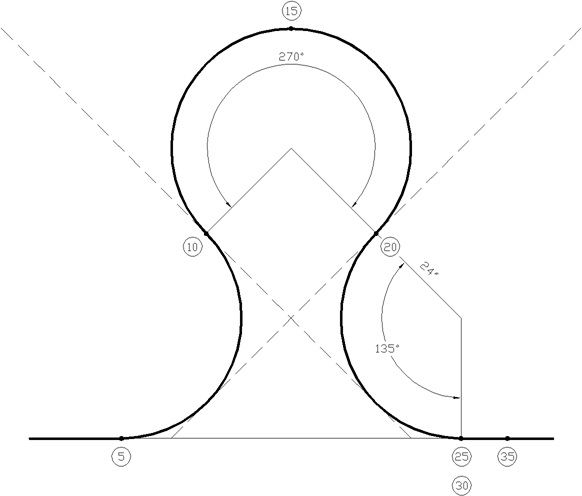Equation 3: Figure 3

Below is an input listing for the model. The delta dimensions shown were obtained from Figure 1. Three additional, equally-spaced points are located on each bend.This example requires a change in the CAESAR II Configuration Editor to allow the error checker to accept large angle (> 95°) bends. \

Click Tools > Configure / Setup to open the CAESAR II Configuration Editor. In the Categories pane, click Geometry Directives and set Maximum Allowable Bend Angle to 135.